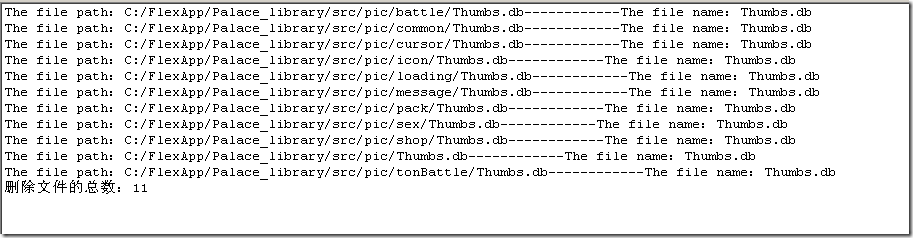# perl删除Windows下的图片缓存缩略图(Thumbs.db)

接手以前别人做的项目，发现SVN里的ignore里并没有对*.db处理，导致图片的缓存缩略文件都被提交了，而我只要打开图片文件夹，就意味着Thumbs.db发生了改变。

`#!/usr/bin/perl`
`use strict;`
`use warnings;`
` `
`my \$path = "C:/FlexApp/xx";`
`my \$filecount = 0;`
` `
`sub parse_env {`
`     my \$path = \$_; #或者使用 my(\$path) = @_; @_类似javascript中的arguments`
`     my \$subpath;`
`     my \$handle;`
` `
`     if (-d \$path) {#当前路径是否为一个目录`
`         if (opendir(\$handle, \$path)) {`
`             while (\$subpath = readdir(\$handle)) {`
`                 if (!(\$subpath =~ m/^\.\$/) and !(\$subpath =~ m/^(\.\.)\$/)) {`
`                     my \$p = \$path."/\$subpath";`
` `
`                     if (-d \$p) {`
`                         parse_env(\$p);`
`                     } elsif (\$subpath eq "Thumbs.db") {`
`                         ++\$filecount;`
`                            print "The file path：".\$p."------------The file name：\$subpath\n";`
`                         unlink(\$p) or warn "failed on \$subpath:\$!";`
`                     }`
`                 }`
`             }`
`             closedir(\$handle);`
`         }`
`     }`
` `
`     return \$filecount;`
`}`
` `
`my \$count = parse_env \$path;`
`my \$str = "删除文件的总数：".\$count;`
` `
`print \$str;`